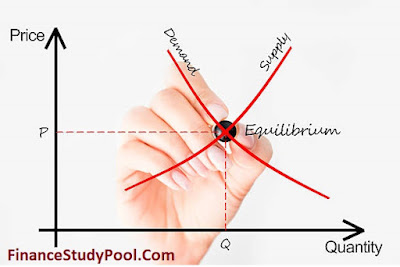# Supply, Demand and Equilibrium Price MCQs - Finance Study Pool

### Supply, Demand and Equilibrium Price

Overview.

The interaction of supply and demand in a market determines the equilibrium price and quantity of a good or service. The equilibrium price is the price at which the quantity supplied equals the quantity demanded, while the equilibrium quantity is the quantity of the good or service that is bought and sold at the equilibrium price Supply, Demand and Equilibrium Price . If the price is too high, there will be a surplus of the good, and producers will need to lower the price to sell their excess supply. Conversely, if the price is too low, there will be a shortage of the good, and consumers will be willing to pay more to obtain it. This will cause producers to increase their prices until the equilibrium is reached.Supply, Demand and Equilibrium Price MCQs

### 1. When the price of goods increases, ceteris paribus:

a) Quantity demanded decreases

b) Quantity supplied decreases

c) Quantity demanded increases

d) Quantity supplied increases

### 2. Increasing income will, ceteris paribus, result in:

a) A decrease in demand for normal goods

b) A decrease in demand for inferior goods

c) An increase in demand for normal goods

d) An increase in demand for inferior goods

### 3. When the price of a substitute good increases, ceteris paribus, we would expect:

a) A decrease in demand for the substitute good

b) An increase in demand for the substitute good

c) A decrease in demand for the complementary good

d) An increase in demand for the complementary good

### 4. Demand for a good must be perfectly elastic if

a) The demand curve is vertical

b) The demand curve is horizontal

c) The quantity demanded is insensitive to changes in price

d) The quantity demanded is very sensitive to changes in price

### 5. When the price of goods increases, ceteris paribus, producers will:

a) Increase their supply of the good

b) Decrease their supply of the good

c) Increase their demand for the good

d) Decrease their demand for the good

### 6. What is the term used to describe the satisfaction a consumer derives from consuming a good or service?

a) Utility

b) Marginal benefit

c) Marginal cost

d) Total cost

### 7. Which of the following is an assumption of the consumer equilibrium model?

a) Consumers have unlimited income

b) Consumers have perfect information about prices and quantities

c) Consumers only care about the price

d) Consumers have a limited budget

### 8. The point where the budget constraint and indifference curve intersect is called:

a) Consumer equilibrium

b) Marginal utility

c) Marginal cost

d) Marginal revenue

### 9. Which of the following statements is accurate when a customer finds equilibrium?

a) The consumer's budget is fully spent

b) The consumer has achieved the maximum possible level of utility

c) The consumer has no further needs or wants

d) The consumer has purchased all of the goods available in the market

Answer: b) The consumer has achieved the maximum possible level of utility{alertSuccess}

### 10. Which of the following factors can shift a consumer's budget constraint?

a) Changes in income

b) Changes in price

c) Changes in tastes and preferences

d) Changes in technology

### 11. Which of the following is the correct formula for calculating marginal utility?

a) Change in the amount demanded or the price

b) Change in total utility/change in quantity consumed

c) Change in total utility/change in price

d) Change in quantity consumed / change in income

### 12. Which of the following is the law of diminishing marginal utility?

a) As the price of goods increases, the quantity demanded decreases

b) As a consumer's income increases, the demand for normal goods increases

c) As a consumer consumes more of a good, the marginal utility of that good decreases

d) As the price of goods decreases, the quantity demanded increases

Answer: c) As a consumer consumes more of a good, the marginal utility of that good decreases{alertSuccess}

### 13. Total utility Vs marginal utility?

a) Total utility is the satisfaction a consumer derives from consuming a good, while marginal utility is the satisfaction derived from consuming an additional unit of that good

b) Total utility is the satisfaction derived from consuming an additional unit of a good, while marginal utility is the satisfaction derived from consuming all units of a good

c) Total utility is the amount of a good consumed, while the marginal utility is the amount of money spent on that good

d) Total utility is the amount of money spent on a good, while the marginal utility is the amount of a good consumed

Answer: a) Total utility is the satisfaction a consumer derives from consuming a good, while marginal utility is the satisfaction derived from consuming an additional unit of that good{alertSuccess}

### 14. Which of the following is the formula for calculating the marginal rate of substitution?

a) Change in price of good Y / Change in quantity of good X

b) Change in quantity of good X / Change in quantity of good Y

c) Change in total utility of good Y / Change in total utility of good X

d) Change in the marginal utility of good X / Change in the marginal utility of good Y

Answer: d) Change in the marginal utility of good X / Change in the marginal utility of good Y{alertSuccess}

M.A Jinnah

As an Editor-in-Chief of financestudypool.com, my role is to supervise the website’s content creation, management, and publication process.